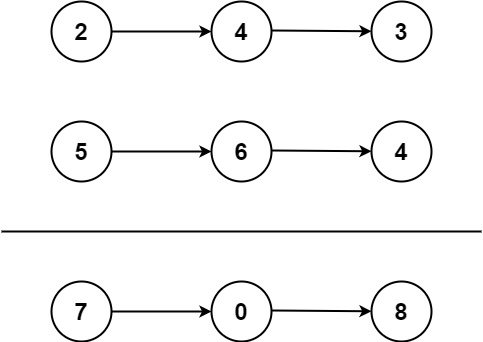### LeetCode• ㊗️
• 大家
• offer
• 多多！

## Problem

You are given two non-empty linked lists representing two non-negative integers. The digits are stored in reverse order and each of their nodes contain a single digit. Add the two numbers and return it as a linked list.

You may assume the two numbers do not contain any leading zero, except the number 0 itself.

Example 1:Input: l1 = [2,4,3], l2 = [5,6,4]
Output: [7,0,8]
Explanation: 342 + 465 = 807.


Example 2:

Input: l1 = , l2 = 
Output: 


Example 3:

Input: l1 = [9,9,9,9,9,9,9], l2 = [9,9,9,9]
Output: [8,9,9,9,0,0,0,1]


Constraints:

• The number of nodes in each linked list is in the range [1, 100].
• 0 <= Node.val <= 9
• It is guaranteed that the list represents a number that does not have leading zeros.

## Code

class Solution {
public ListNode addTwoNumbers(ListNode l1, ListNode l2) {
ListNode dummy = new ListNode(-1);
ListNode curr = dummy;

int carry = 0;
while(l1 != null || l2 != null){
int x = l1 == null ? 0 : l1.val;
int y = l2 == null ? 0 : l2.val;

int sum = x + y + carry;
curr.next = new ListNode(sum % 10);
carry = sum / 10;
curr = curr.next;

if(l1 != null) l1 = l1.next;
if(l2 != null) l2 = l2.next;
}

if(carry != 0){
curr.next = new ListNode(carry);
}
return dummy.next;
}
}

class Solution:
def addTwoNumbers(self, l1: ListNode, l2: ListNode) -> ListNode:
dummy = ListNode(-1)
curr = dummy

carry = 0
while l1 or l2:
num1 = l1.val if l1 else 0
num2 = l2.val if l2 else 0

sum = num1 + num2 + carry
curr.next = ListNode(sum % 10)
curr = curr.next
carry = sum // 10

l1 = l1.next if l1 else None
l2 = l2.next if l2 else None

if carry:
curr.next = ListNode(carry)

return dummy.next